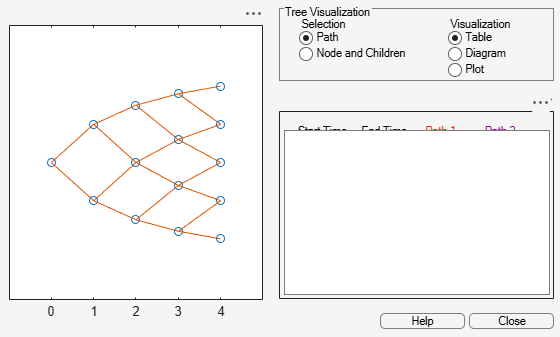# Pricing a Portfolio Using the Black-Derman-Toy Model

This example illustrates how the Financial Instruments Toolbox™ is used to create a Black-Derman-Toy (BDT) tree and price a portfolio of instruments using the BDT model.

### Create the Interest Rate Term Structure

The structure `RateSpec` is an interest-rate term structure that defines the initial forward-rate specification from which the tree rates are derived. Use the information of annualized zero coupon rates in the table below to populate the `RateSpec` structure.

` From To Rate`

`01 Jan 2005 01 Jan 2006 0.0275`

`01 Jan 2005 01 Jan 2007 0.0312`

`01 Jan 2005 01 Jan 2008 0.0363`

`01 Jan 2005 01 Jan 2009 0.0415`

`01 Jan 2005 01 Jan 2010 0.0458`

```StartDates = ['01 Jan 2005']; EndDates = ['01 Jan 2006'; '01 Jan 2007'; '01 Jan 2008'; '01 Jan 2009'; '01 Jan 2010']; ValuationDate = ['01 Jan 2005']; Rates = [0.0275; 0.0312; 0.0363; 0.0415; 0.0458]; Compounding = 1; RateSpec = intenvset('Compounding',Compounding,'StartDates', StartDates,... 'EndDates', EndDates, 'Rates', Rates,'ValuationDate', ValuationDate)```
```RateSpec = struct with fields: FinObj: 'RateSpec' Compounding: 1 Disc: [5x1 double] Rates: [5x1 double] EndTimes: [5x1 double] StartTimes: [5x1 double] EndDates: [5x1 double] StartDates: 732313 ValuationDate: 732313 Basis: 0 EndMonthRule: 1 ```

### Specify the Volatility Model

Create the structure `VolSpec` that specifies the volatility process with the following data.

```Volatility = [0.005; 0.0055; 0.006; 0.0065; 0.007]; BDTVolSpec = bdtvolspec(ValuationDate, EndDates, Volatility)```
```BDTVolSpec = struct with fields: FinObj: 'BDTVolSpec' ValuationDate: 732313 VolDates: [5x1 double] VolCurve: [5x1 double] VolInterpMethod: 'linear' ```

### Specify the Time Structure of the Tree

The structure `TimeSpec` specifies the time structure for an interest-rate tree. This structure defines the mapping between the observation times at each level of the tree and the corresponding dates.

```Maturity = EndDates; BDTTimeSpec = bdttimespec(ValuationDate, Maturity, Compounding)```
```BDTTimeSpec = struct with fields: FinObj: 'BDTTimeSpec' ValuationDate: 732313 Maturity: [5x1 double] Compounding: 1 Basis: 0 EndMonthRule: 1 ```

### Create the BDT Tree

Use the previously computed values for `RateSpec`, `VolSpec`, and `TimeSpec` to create the `BDTTree`.

`BDTTree = bdttree(BDTVolSpec, RateSpec, BDTTimeSpec)`
```BDTTree = struct with fields: FinObj: 'BDTFwdTree' VolSpec: [1x1 struct] TimeSpec: [1x1 struct] RateSpec: [1x1 struct] tObs: [0 1 2 3 4] dObs: [732313 732678 733043 733408 733774] TFwd: {[5x1 double] [4x1 double] [3x1 double] [2x1 double] } CFlowT: {[5x1 double] [4x1 double] [3x1 double] [2x1 double] } FwdTree: {1x5 cell} ```

### Observe the Interest Rate Tree

Visualize the interest-rate evolution along the tree by looking at the output structure `BDTTree`. `BDTTree` returns an inverse discount tree, which you can convert into an interest-rate tree with the `cvtree` function.

`BDTTreeR = cvtree(BDTTree);`

Look at the upper branch and lower branch paths of the tree:

```%Rate at root node: RateRoot = treepath(BDTTreeR.RateTree, ) ```
```RateRoot = 0.0275 ```
```%Rates along upper branch: RatePathUp = treepath(BDTTreeR.RateTree, [1 1 1 1]) ```
```RatePathUp = 5×1 0.0275 0.0347 0.0460 0.0560 0.0612 ```
```%Rates along lower branch: RatePathDown = treepath(BDTTreeR.RateTree, [2 2 2 2])```
```RatePathDown = 5×1 0.0275 0.0351 0.0472 0.0585 0.0653 ```

You can also display a graphical representation of the tree to examine interactively the rates on the nodes of the tree until maturity. The function `treeviewer` displays the structure of the rate tree in the left pane. The tree visualization in the right pane is blank, but by selecting `Diagram` and clicking on the nodes you can examine the rates along the paths.

`treeviewer(BDTTreeR)`### Create an Instrument Portfolio

Create a portfolio consisting of two bond instruments and a option on the 5% Bond.

```% Bonds CouponRate = [0.04;0.05]; Settle = '01 Jan 2005'; Maturity = ['01 Jan 2009';'01 Jan 2010']; Period = 1; % Option OptSpec = {'call'}; Strike = 98; ExerciseDates = ['01 Jan 2010']; AmericanOpt = 1; InstSet = instadd('Bond',CouponRate, Settle, Maturity, Period); InstSet = instadd(InstSet,'OptBond', 2, OptSpec, Strike, ExerciseDates, AmericanOpt);```

Examine the set of instruments contained in the variable `InstSet`.

`instdisp(InstSet)`
```Index Type CouponRate Settle Maturity Period Basis EndMonthRule IssueDate FirstCouponDate LastCouponDate StartDate Face 1 Bond 0.04 01-Jan-2005 01-Jan-2009 1 0 1 NaN NaN NaN NaN 100 2 Bond 0.05 01-Jan-2005 01-Jan-2010 1 0 1 NaN NaN NaN NaN 100 Index Type UnderInd OptSpec Strike ExerciseDates AmericanOpt 3 OptBond 2 call 98 01-Jan-2010 1 ```

### Price the Portfolio Using a BDT Tree

Calculate the price of each instrument in the instrument set (`InstSet`) using `bdtprice`.

`Price = bdtprice(BDTTree, InstSet)`
```Price = 3×1 99.6374 102.2460 4.2460 ```

The prices in the output vector `Price` correspond to the prices at observation time zero (`tObs` = `0`), which is defined as the Valuation Date of the interest-rate tree.

In the `Price` vector, the first element, `99.6374`, represents the price of the first instrument (4% Bond); the second element, `102.2460`, represents the price of the second instrument (5% Bond), and `4.2460` represents the price of the Option.